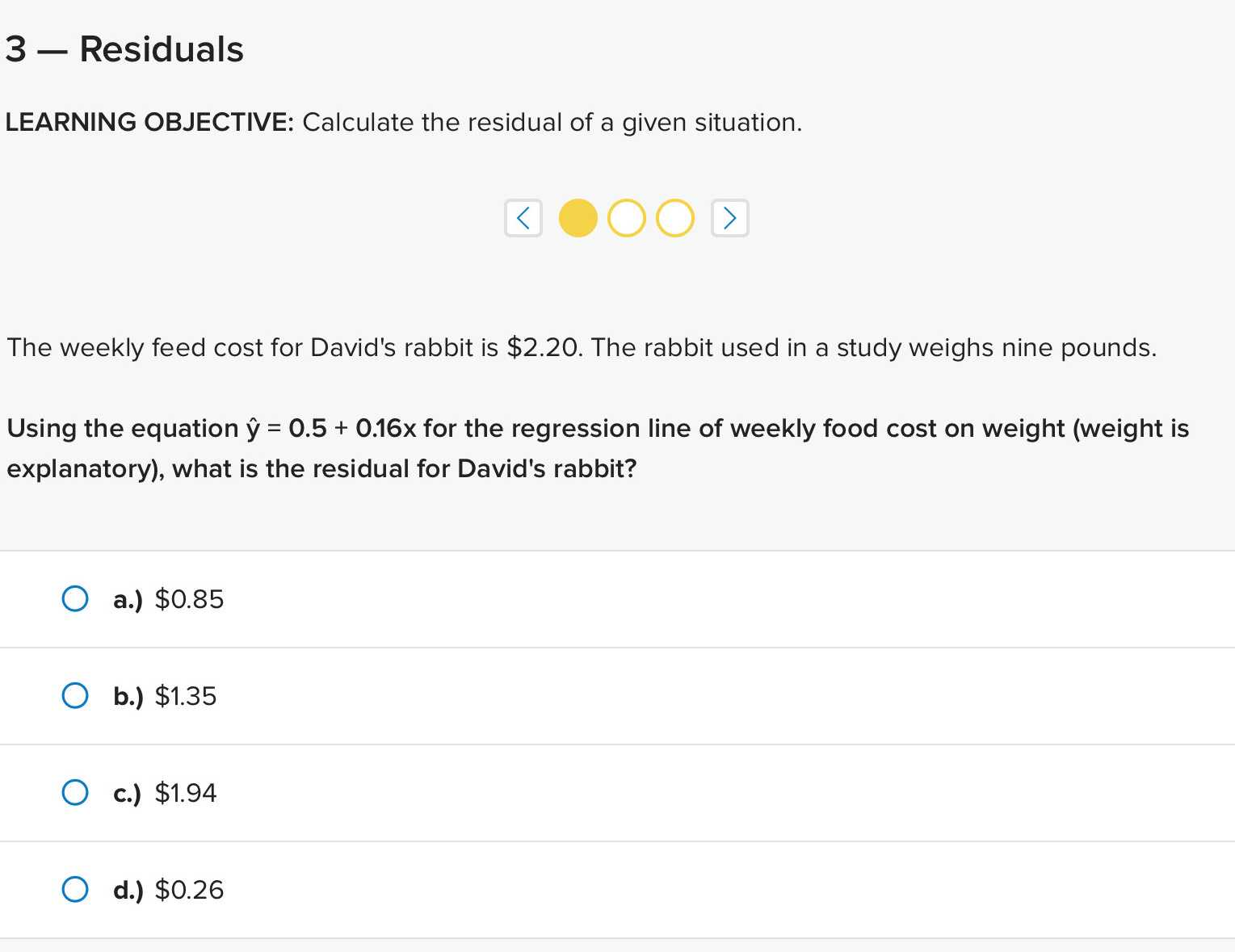### ¿Todavía tienes preguntas de matemáticas?

Pregunte a nuestros tutores expertos
Algebra
Pregunta$$3$$ - Residuals LEARNING OBJECTIVE: Calculate the residual of a given situation. The weekly feed cost for David's rabbit is $$\ 2.20$$ . The rabbit used in a study weighs nine pounds. Using the equation ŷ $$= 0.5 + 0.16 x$$ for the regression line of weekly food cost on weight (weight is explanatory), what is the residual for David's rabbit? a.) $$\ 0.85$$

b.) $$\ 1.35$$

c.) $$\ 1.94$$

d. $$\ 0.26$$